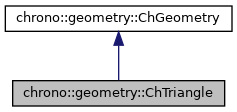chrono::geometry::ChTriangle Class Reference

## Description

A triangle geometric shape for collisions and visualization.

#include <ChTriangle.h>

Inheritance diagram for chrono::geometry::ChTriangle:[legend]
Collaboration diagram for chrono::geometry::ChTriangle:[legend]

## Public Member Functions

ChTriangle (const ChVector<> &mp1, const ChVector<> &mp2, const ChVector<> &mp3)

ChTriangle (const ChTriangle &source)

virtual ChTriangleClone () const override
"Virtual" copy constructor (covariant return type).

ChTriangleoperator= (const ChTriangle &source)
Assignment operator: copy from another triangle.

virtual GeometryType GetClassType () const override
Get the class type as unique numerical ID (faster than using ChronoRTTI mechanism). More...

virtual void GetBoundingBox (ChVector<> &cmin, ChVector<> &cmax, const ChMatrix33<> &rot) const override
Compute bounding box along the directions defined by the given rotation matrix.

virtual ChVector Baricenter () const override
Compute center of mass.

virtual int GetManifoldDimension () const override
This is a surface.

bool IsDegenerated () const

bool Normal (ChVector<> &N) const

ChVector GetNormal () const

double PointTriangleDistance (ChVector<> B, double &mu, double &mv, bool &is_into, ChVector<> &Bprojected)
Given point B, computes the distance from this triangle plane, returning also the projection of point on the plane. More...

virtual void ArchiveOUT (ChArchiveOut &marchive) override
Method to allow serialization of transient data to archives.

virtual void ArchiveIN (ChArchiveIn &marchive) override
Method to allow de-serialization of transient data from archives.Public Member Functions inherited from chrono::geometry::ChGeometry
ChGeometry (const ChGeometry &source)

void InflateBoundingBox (ChVector<> &cmin, ChVector<> &cmax, const ChMatrix33<> &rot) const
Enlarge the given existing bounding box with the bounding box of this object.

Returns the radius of a bounding sphere for this geometry. More...

virtual void Update ()
Generic update of internal data.

## Public Attributes

ChVector p1
first triangle vertex

ChVector p2
second triangle vertex

ChVector p3
third triangle vertexPublic Types inherited from chrono::geometry::ChGeometry
enum  GeometryType {
NONE, SPHERE, ELLIPSOID, BOX,
CYLINDER, TRIANGLE, CAPSULE, CONE,
LINE, LINE_ARC, LINE_BEZIER, LINE_CAM,
LINE_PATH, LINE_POLY, LINE_SEGMENT, ROUNDED_BOX,
ROUNDED_CYLINDER, ROUNDED_CONE, TRIANGLEMESH, TRIANGLEMESH_CONNECTED,
TRIANGLEMESH_SOUP
}
Enumeration of geometric object types.

## ◆ GetClassType()

 virtual GeometryType chrono::geometry::ChTriangle::GetClassType ( ) const
inlineoverridevirtual

Get the class type as unique numerical ID (faster than using ChronoRTTI mechanism).

Each inherited class must return an unique ID.

Reimplemented from chrono::geometry::ChGeometry.

## ◆ PointTriangleDistance()

 double chrono::geometry::ChTriangle::PointTriangleDistance ( ChVector<> B, double & mu, double & mv, bool & is_into, ChVector<> & Bprojected )

Given point B, computes the distance from this triangle plane, returning also the projection of point on the plane.

Parameters
 B point to be measured mu returns U parametric coord of projection mv returns V parametric coord of projection is_into returns true if projection falls on the triangle Bprojected returns the position of the projected point

The documentation for this class was generated from the following files:
• /builds/uwsbel/chrono/src/chrono/geometry/ChTriangle.h
• /builds/uwsbel/chrono/src/chrono/geometry/ChTriangle.cpp